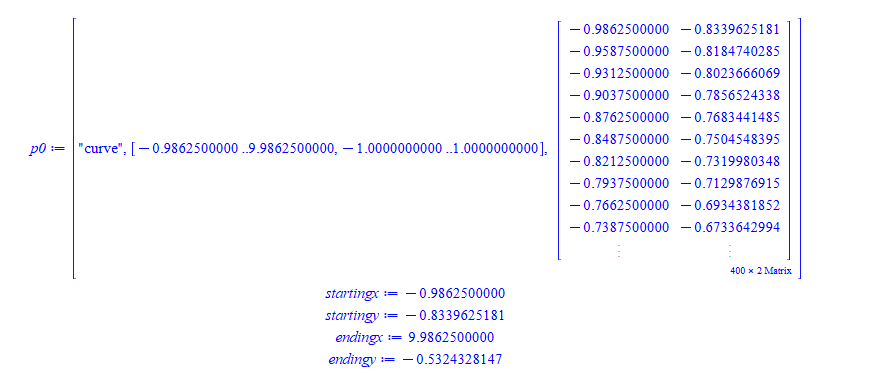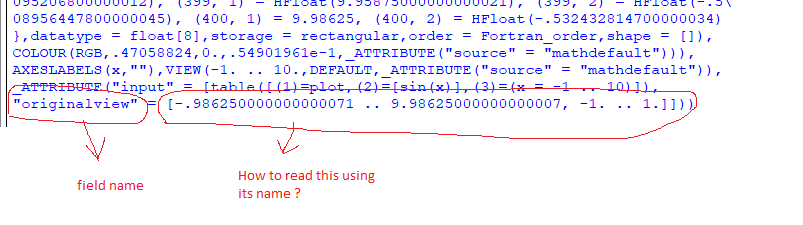# Question:how to read the "originalview" or the range of x and y used in generating a 2D plot?

## Question:how to read the "originalview" or the range of x and y used in generating a 2D plot?

Maple 2022

I create a plot, then later on, I want to use the same view for a second plot or using the same starting x, ending x and starting y and ending y. For reasons no need to get into into, I need to do this and I have no access to this information at this time. I only have access to the p variable used to store the plot into as in this example below.

I am not sure what is the correct way to do read this information from the PLOTS structure as I never used it before.

Help describes the PLOTS structure under "PLOT and PLOT3D Data Structures" , but do not see how is one supposed to read different parts of it. I could have missed it. Maple help pages are very hard for me to read in order to obtain the information needed. From help it says:

The Maple plotting functions, plot, plot3d, and others, produce PLOT and PLOT3D data structures describing the images to be displayed.

By trial and error, for standard plot this worked

```p:=plot(sin(x),x=-1..10):
op([4,1,2,2],p)
```

returns

"originalview" = [-0.9862500000 .. 9.9862500000, -1.0000000000 .. 1.0000000000]

I see also that command plottools:-getdata(p):  returns the data. So I could also do (it is stored in matrix, in third entry):

```p0:=plottools:-getdata(p);
startingx:=p0[1,1];
startingy:=p0[1,2];
endingx:=p0[-1,1];
endingy:=p0[-1,2];

```Will the above work all the time? should this command be used to obtain this information or is there a better way?

When I lprint(p) I see these fields have actual names. For example  , here is screen shot of lprint(p)it looks like  a RECORD data structure. But when I tried p:-originalview  Error, `p` does not evaluate to a module

The question is: What is the correct way to find what x range and y range was used for an earlier plot, given one has access only to p variable used to store the output of the plot command?  This is for 2D plot only.﻿